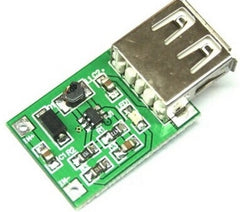# 📖 DC-DC converters guide

DC / DC converters are widely used for powering various electronic equipment. They are used in computing devices, communication devices, various control, and automation schemes, etc.

Transformer Power Supplies

In traditional transformer power units, the supply voltage is transformed by the transformer, most often lowered, to the desired value. The reduced voltage is rectified by a diode bridge and smoothed by a capacitor filter. If necessary, a semiconductor stabilizer is placed after the rectifier.

Transformer power supplies, as a rule, are equipped with linear stabilizers. The advantages of such stabilizers are at least two: this is a small cost and a small number of parts in the harness. But these advantages are eaten by low efficiency since a significant part of the input voltage is used to heat the control transistor, which is completely unacceptable for powering portable electronic devices.

DC / DC converters

If the equipment is powered from galvanic cells or batteries, then voltage conversion to the desired level is possible only with the help of DC / DC converters.

The idea is quite simple: DC voltage is converted to AC, usually with a frequency of several tens or even hundreds of kilohertz, rises (decreases), and then straightens and is fed to the load. Such converters are often called impulse.

As an example, we can take a boost converter from 1.5V to 5V, just the output voltage of a computer USB. Such a converter of low power is sold to Aliexpress.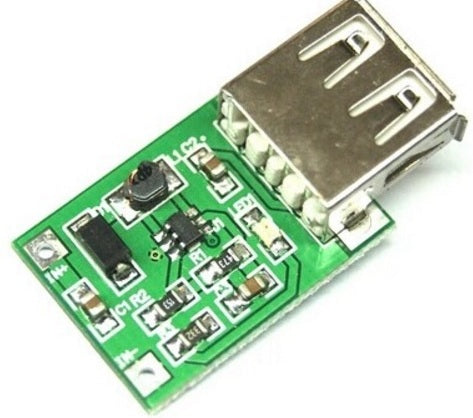Pulse converters are good because they have high efficiency, within 60..90%. Another advantage of pulse converters is a wide range of input voltages: the input voltage can be lower than the output voltage or much higher. In general, DC / DC converters can be divided into several groups.

Converter classification

Step-down or buck lowering

The output voltage of these converters, as a rule, is lower than the input one: without any special heat losses of the regulating transistor, you can get a voltage of only a few volts at an input voltage of 12 ... 50V. The output current of such converters depends on the needs of the load, which in turn determines the converter circuitry.

Another English name for the chopper down converter. One of the translations for this word is a chopper. In technical literature, a down converter is sometimes called a “chopper.” For now, just remember this term.

Boost, in English terminology step-up or boost

The output voltage of these converters is higher than the input. For example, at an input voltage of 5V, the output can receive voltages up to 30V, moreover, it may be smoothly regulated and stabilized. Quite often, up-converters are called boosters.

Universal Converters - SEPIC

The output voltage of these converters is held at a predetermined level at an input voltage both above the input and below. It is recommended in cases where the input voltage can vary significantly. For example, in a car, the battery voltage may vary within 9 ... 14V and a stable voltage of 12V is required.

Inverting converters - inverting converter

The main function of these converters is to obtain at the output voltage of reverse polarity relative to the power supply. It is very convenient in those cases when bipolar power is required, for example, to power an opamp.

All of the mentioned converters can be stabilized or unstabilized, the output voltage can be galvanically connected to the input or be galvanically isolated from the voltages. It all depends on the specific device in which the converter will be used.

In order to proceed to the further story about DC / DC converters, you should at least in general terms understand the theory.

Chopper down converter - buck type converter

Its functional diagram is shown in the figure below. The arrows on the wires show the direction of the currents.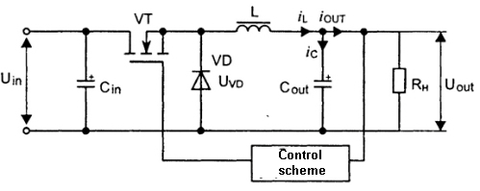The input voltage Uin is fed to the input filter - capacitor Cin. The transistor VT is used as a key element; it performs high-frequency switching of the current. This may be a MOSFET transistor, IGBT structure or a conventional bipolar transistor. In addition to these parts, the circuit contains a bit diode VD and an output filter - LCout, from which the voltage enters the load RL.

It is easy to see that the load is connected in series with the elements VT and L. Therefore, the circuit is sequential. How does the voltage drop?

Pulse Width Modulation - PWM

The control circuit generates rectangular pulses with a constant frequency or a constant period, which is essentially the same. These pulses are shown in Figure 3.Here ti is the pulse time, the transistor is open, tn is the pause time, the transistor is closed. The ratio ti / T is called the duty cycle, denoted by the letter D and expressed in %% or simply in numbers. For example, when D is 50%, it turns out that D = 0.5.

Thus, D can vary from 0 to 1. When D = 1, the key transistor is in the state of full conductivity, and when D = 0, in the cutoff state, simply speaking, it is closed. It is not hard to guess that at D = 50% the output voltage will be equal to half the input.

It is obvious that the regulation of the output voltage occurs due to a change in the width of the control pulse t and, in fact, a change in the coefficient D. This regulation principle is called pulse-width modulation PWM. Practically in all switching power supply units, it is with the help of PWM that the output voltage is stabilized.

In the diagrams shown in Figures 2 and 6, the PWM is “hidden” in rectangles labeled “Control Circuit”, which performs some additional functions. For example, it may be a smooth start of the output voltage, remote switching on or protection of the converter from a short circuit.

In general, the converters are so widely used that the manufacturers of electronic components have established the release of PWM controllers for all occasions. The range is so large that just in order to list them you will need a whole book. Therefore, collecting converters on discrete elements, or as they often say on the “wastage”, does not occur to anyone.

Moreover, ready-made low-power converters can be bought on Aliexpress or Ebay for a small price. At the same time, for installation in the amateur construction, it is enough to solder the wires to the board for input and output and set the required output voltage.

But back to our picture 3. In this case, the coefficient D determines how long the key transistor will be open (phase 1) or closed (phase 2). For these two phases, it is possible to present a diagram in two figures. The figures do not show those elements that are not used in this phase.When the transistor is open, the current from the power source (galvanic cell, battery, rectifier) ​​passes through inductive choke L, load Rн, and charging capacitor Cout. In this case, a current flows through the load, the capacitor Cout and the choke L accumulate energy. The current iL GRADUALLY GROWTH is affected by the influence of the inductor inductance. This phase is called pumping.

After the voltage on the load reaches a predetermined value (determined by the setting of the control device), the transistor VT closes and the device switches to the second phase - the discharge phase. The closed transistor in the figure is not shown at all, as if it is not. But this only means that the transistor is closed.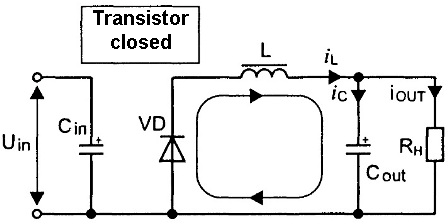When the transistor VT is closed, energy is not replenished in the choke because the power supply is disconnected. Inductance L tends to prevent a change in the magnitude and direction of the current (self-induction) of the choke flowing through the winding.

Therefore, the current can not instantly stop and closes through the "diode-load" circuit. Because of this, the diode VD was named bit. Typically, this is a high-speed Schottky diode. After the control period expires, phase 2 of the circuit switches to phase 1, the process is repeated again. The maximum voltage at the output of the considered circuit can be equal to the input, and no more. To get the output voltage greater than the input, step-up converters are used.

It should be noted that in reality everything is not as simple as it is written above: it is assumed that all components are ideal, i.e. on and off occurs without delay, and the resistance is zero. In the practical manufacture of such schemes, many nuances have to be taken into account, since very much depends on the quality of the components used and the parasitic mounting capacity. Only about such a simple detail as a choke (well, just a bundle of wire!) You can write more than one article.

So far it should only be recalled of the inductance itself, which determines the two modes of operation of the chopper. With insufficient inductance, the converter will operate in the mode of discontinuous currents, which is completely unacceptable for power supplies.

If the inductance is large enough, then the work takes place in the mode of continuous currents, which allows using the output filters to obtain a constant voltage with an acceptable level of ripple. In the mode of continuous currents work and step-up converters, which will be discussed below.

For a certain increase in efficiency, the discharge diode VD is replaced by a MOSFET transistor, which is opened at the right moment by the control circuit. Such converters are called synchronous. Their use is justified if the converter power is large enough.

Step-up or boost converters

Boost converters are used mainly with low-voltage power supply, for example, from two to three batteries, and some components of the design require a voltage of 12 ... 15 V with low current consumption. Quite often, the boost converter is briefly and clearly called the word "booster."The input voltage Uin is applied to the input filter Cin and is applied to the series-connected inductor L and the switching transistor VT. A diode VD is connected to the connection point of the coil and drain of the transistor. To another pin of the diode, the load Rh and the shunt capacitor Cout are connected.

The VT transistor is controlled by a control circuit that produces a stable frequency control signal with an adjustable duty cycle D, just as described above in describing the chopper circuit (Fig. 3). Diode VD at the right time blocks the load from the key transistor.

When the key transistor is open, right according to the scheme, the output of the coil L is connected to the negative pole of the power source Uin. The increasing current (the effect of inductance effects) from the power source flows through the coil and the open transistor, energy is accumulated in the coil.

At this time, the diode VD blocks the load and the output capacitor from the key circuit, thereby preventing the discharge of the output capacitor through the open transistor. The load at this moment is powered by the energy stored in the capacitor Cout. Naturally, the voltage on the output capacitor drops.

As soon as the output voltage is slightly lower than the preset (determined by the settings of the control circuit), the key transistor VT closes and the energy stored in the inductor through the diode VD charges the capacitor Cout, which feeds the load. In this case, the self-induction EMF of the coil L is added to the input voltage and transferred to the load, therefore, the output voltage is greater than the input voltage.

When the output voltage reaches the set stabilization level, the control circuit opens the transistor VT, and the process repeats from the energy accumulation phase.

Universal converters - SEPIC (single-ended primary-inductor converter or converter with asymmetrically loaded primary inductance).

Such converters are used mainly when the load has a negligible power, and the input voltage changes relative to the output up or down.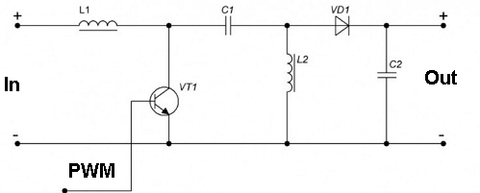It is very similar to the boost converter circuit shown in Figure 6, but it has additional elements: a capacitor C1 and a coil L2. It is these elements that ensure the operation of the converter in the voltage reduction mode.

SEPIC converters are used in cases where the input voltage varies widely. As an example, 4V-35V to 1.23V-32V Boost Buck Voltage Step Up / Down Converter Regulator. It is under this name in Chinese stores that a converter is sold, the circuit of which is shown in Figure 8 (click on image to enlarge).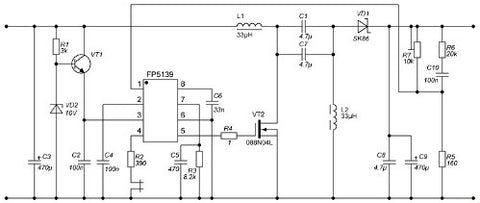Figure 9 shows the appearance of the board with the designation of the main elements.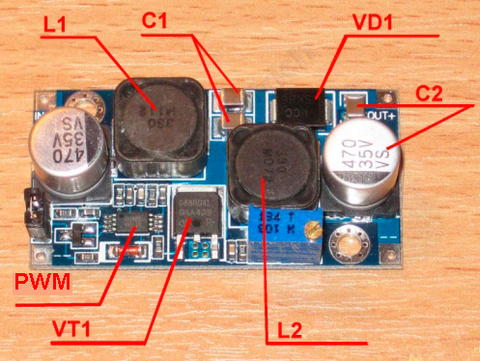The figure shows the main parts in accordance with Figure 7. Attention should be paid to the presence of two coils L1 L2. On this basis, it is possible to determine that this is the SEPIC converter.

The input voltage of the board can be within 4 ... 35V. In this case, the output voltage can be adjusted in the range of 1.23 ... 32V. The operating frequency of the inverter is 500 KHz. In the case of small dimensions of 50 x 25 x 12 mm, the board provides power up to 25 W. Maximum output current up to 3A.

But here it is necessary to make a remark. If the output voltage is set at 10V, then the output current cannot be higher than 2.5A (25W). With an output voltage of 5V and a maximum current of 3A, the power will be only 15W. The main thing here is not to overdo it: either do not exceed the maximum allowable power or do not go beyond the limits of the allowable current.